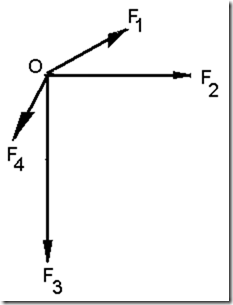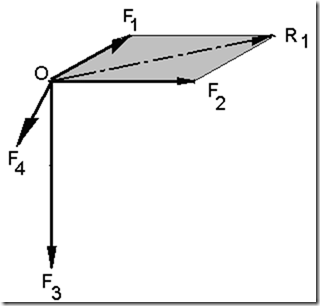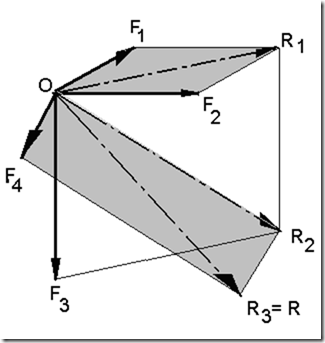Home > English > Add Style With Painting

# Add Style With Painting

Develop or add styles that have more force than the two styles with the same capture point the way can be described by the following figure:
In preparation for painting style of drawing tools necessary tool especially crossbar triangular one pair and stationery or engineering techniques using pencil and ruler triangle one pair of which is to create a line of parallel lines.

Draw a picture style component which consists of four styles have a different direction with the same capture point as shown below, adjusted to the length of the line style scale.Figure 2.18 adds style by means of a parallelogram

Sum of F1 to F2 by means of a parallelogram, use a ruler to draw a line a new set of alignment lines. thus obtained F1 + F2 = R1, as shown in the following figure.Figure 2.19 adds style F1 + F2 = R1

Sum of F1 + F2 + F3 = R2 or R1 + F3 = R2. by means of a parallelogram as above. See the picture below!Figure 2.20 adds style F1 + F2 + F3 = R2 or R1 + F3 = R2

Sum of F1 + F2 + F3 + F4 = R, or R1 + R2 + F4 = R3 = R by means of a parallelogram as above. See the picture below!Figure 2.21 sum F1 + F2 + F3 + F4 = R"Tasikmalaya, Indonesia"